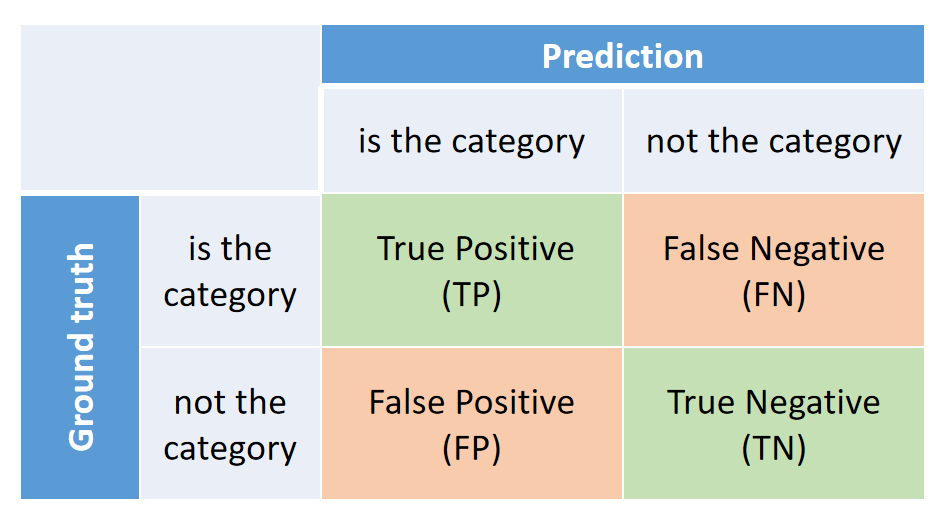Shortcuts

# SingleLabelMetric¶

class mmpretrain.evaluation.SingleLabelMetric(thrs=0.0, items=('precision', 'recall', 'f1-score'), average='macro', num_classes=None, collect_device='cpu', prefix=None)[源代码]

A collection of precision, recall, f1-score and support for single-label tasks.

The collection of metrics is for single-label multi-class classification. And all these metrics are based on the confusion matrix of every category:All metrics can be formulated use variables above:

Precision is the fraction of correct predictions in all predictions:

$\text{Precision} = \frac{TP}{TP+FP}$

Recall is the fraction of correct predictions in all targets:

$\text{Recall} = \frac{TP}{TP+FN}$

F1-score is the harmonic mean of the precision and recall:

$\text{F1-score} = \frac{2\times\text{Recall}\times\text{Precision}}{\text{Recall}+\text{Precision}}$

Support is the number of samples:

$\text{Support} = TP + TN + FN + FP$

• thrs (Sequence[float | None] | float | None) – If a float, predictions with score lower than the threshold will be regard as the negative prediction. If None, only the top-1 prediction will be regard as the positive prediction. If the parameter is a tuple, accuracy based on all thresholds will be calculated and outputted together. Defaults to 0.

• items (Sequence[str]) – The detailed metric items to evaluate, select from “precision”, “recall”, “f1-score” and “support”. Defaults to ('precision', 'recall', 'f1-score').

• average (str | None) –

How to calculate the final metrics from the confusion matrix of every category. It supports three modes:

• ”macro”: Calculate metrics for each category, and calculate the mean value over all categories.

• ”micro”: Average the confusion matrix over all categories and calculate metrics on the mean confusion matrix.

• None: Calculate metrics of every category and output directly.

Defaults to “macro”.

• num_classes (int, optional) – The number of classes. Defaults to None.

• collect_device (str) – Device name used for collecting results from different ranks during distributed training. Must be ‘cpu’ or ‘gpu’. Defaults to ‘cpu’.

• prefix (str, optional) – The prefix that will be added in the metric names to disambiguate homonymous metrics of different evaluators. If prefix is not provided in the argument, self.default_prefix will be used instead. Defaults to None.

>>> import torch
>>> from mmpretrain.evaluation import SingleLabelMetric
>>> # -------------------- The Basic Usage --------------------
>>> y_pred = [0, 1, 1, 3]
>>> y_true = [0, 2, 1, 3]
>>> # Output precision, recall, f1-score and support.
>>> SingleLabelMetric.calculate(y_pred, y_true, num_classes=4)
(tensor(62.5000), tensor(75.), tensor(66.6667), tensor(4))
>>> # Calculate with different thresholds.
>>> y_score = torch.rand((1000, 10))
>>> y_true = torch.zeros((1000, ))
>>> SingleLabelMetric.calculate(y_score, y_true, thrs=(0., 0.9))
[(tensor(10.), tensor(0.9500), tensor(1.7352), tensor(1000)),
(tensor(10.), tensor(0.5500), tensor(1.0427), tensor(1000))]
>>>
>>> # ------------------- Use with Evalutor -------------------
>>> from mmpretrain.structures import DataSample
>>> from mmengine.evaluator import Evaluator
>>> data_samples = [
...     DataSample().set_gt_label(i%5).set_pred_score(torch.rand(5))
...     for i in range(1000)
... ]
>>> evaluator = Evaluator(metrics=SingleLabelMetric())
>>> evaluator.process(data_samples)
>>> evaluator.evaluate(1000)
{'single-label/precision': 19.650691986083984,
'single-label/recall': 19.600000381469727,
'single-label/f1-score': 19.619548797607422}
>>> # Evaluate on each class
>>> evaluator = Evaluator(metrics=SingleLabelMetric(average=None))
>>> evaluator.process(data_samples)
>>> evaluator.evaluate(1000)
{
'single-label/precision_classwise': [21.1, 18.7, 17.8, 19.4, 16.1],
'single-label/recall_classwise': [18.5, 18.5, 17.0, 20.0, 18.0],
'single-label/f1-score_classwise': [19.7, 18.6, 17.1, 19.7, 17.0]
}

static calculate(pred, target, thrs=(0.0,), average='macro', num_classes=None)[源代码]

Calculate the precision, recall, f1-score and support.

• pred (torch.Tensor | np.ndarray | Sequence) – The prediction results. It can be labels (N, ), or scores of every class (N, C).

• target (torch.Tensor | np.ndarray | Sequence) – The target of each prediction with shape (N, ).

• thrs (Sequence[float | None]) – Predictions with scores under the thresholds are considered negative. It’s only used when pred is scores. None means no thresholds. Defaults to (0., ).

• average (str | None) –

How to calculate the final metrics from the confusion matrix of every category. It supports three modes:

• ”macro”: Calculate metrics for each category, and calculate the mean value over all categories.

• ”micro”: Average the confusion matrix over all categories and calculate metrics on the mean confusion matrix.

• None: Calculate metrics of every category and output directly.

Defaults to “macro”.

• num_classes (Optional, int) – The number of classes. If the pred is label instead of scores, this argument is required. Defaults to None.

The tuple contains precision, recall and f1-score. And the type of each item is:

• torch.Tensor: If the pred is a sequence of label instead of score (number of dimensions is 1). Only returns a tensor for each metric. The shape is (1, ) if classwise is False, and (C, ) if classwise is True.

• List[torch.Tensor]: If the pred is a sequence of score (number of dimensions is 2). Return the metrics on each thrs. The shape of tensor is (1, ) if classwise is False, and (C, ) if classwise is True.

Tuple

compute_metrics(results)[源代码]

Compute the metrics from processed results.

results (list) – The processed results of each batch.

The computed metrics. The keys are the names of the metrics, and the values are corresponding results.

Dict

process(data_batch, data_samples)[源代码]

Process one batch of data samples.

The processed results should be stored in self.results, which will be used to computed the metrics when all batches have been processed.

• data_batch – A batch of data from the dataloader.

• data_samples (Sequence[dict]) – A batch of outputs from the model.

© Copyright 2020, OpenMMLab. Revision bb59c9ad.

Built with Sphinx using a theme provided by Read the Docs.